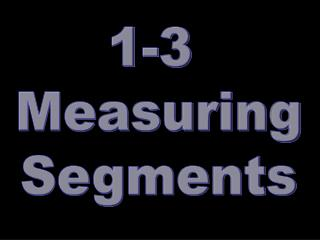DownloadDownload Presentation1-3 Measuring Segments

# 1-3 Measuring Segments

Télécharger la présentation## 1-3 Measuring Segments

- - - - - - - - - - - - - - - - - - - - - - - - - - - E N D - - - - - - - - - - - - - - - - - - - - - - - - - - -
##### Presentation Transcript

1. 1-3 Measuring Segments

2. J A M (1-5 Postulate) Ruler Postulate • COORDINATE – number associated with a letter • Coordinate of M? • Coordinate of J? 7 -4

3. J A M Distance • The distance of a segment = length • JA = ? • AM = ? 6 5

4. Segment Addition Postulate (Postulate 1-6) • If three points A, B and C are collinear and B is between Aand C, then AB + BC = AC A B C

5. If LN = 32, what are LM and MN? 3x + 8 2x + 4 L M N

6. CONGRUENCE • CONGRUENT: two objects that have the same size and shape • Congruent Segments: segments with equal lengths. 12.4 12.4

7. MIDPOINT Midpoint of a Segment: • is the point that divides the segment into twocongruentsegments.

8. BISECTOR Segment Bisector: is a line, segment, ray, or plane that intersects the segment at its midpoint.

9. Find x if RA = 2x + 4, AY = 3x + 1, and RY = 30. Find x if CA = 7x-3, AZ = 3x + 1, and line RY bisects segment CZ. Z 3x + 1 R 2x + 4 A 3x + 1 7x -3 Y 30 2x + 4 + 3x + 1 = 30 C 7x – 3 = 3x + 1 5x + 5 = 30 4x = 4 - 5 - 5 5x = 25 x = 1 /5 /5 x = 5